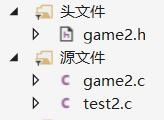# 如何利用C语言实现扫雷小游戏 利用C语言实现扫雷小游戏代码方法test2.c：整个游戏，开始游戏/退出游戏的大体执行流程

game2.c：具体实现扫雷游戏功能的函数定义

game.h：宏定义，函数声明，引用相关C库函数的头文件

test2.c

```#include"game2.h"

//菜单函数
{
printf("**************************n");
printf("******** 1.play ********n");
printf("******** 0.exit ********n");
printf("**************************n");
}

//游戏函数
void game()
{
//雷的信息储存
//1.雷分布的棋盘
char mine[ROWS][COLS] = { 0 };//玩家不能看
//2.排查雷信息的棋盘
char show[ROWS][COLS] = { 0 };//玩家进行游戏时候的棋盘

//初始化棋盘
InitBoard(mine, ROWS, COLS, '0');
InitBoard(show, ROWS, COLS, '*');

//打印棋盘
DisplayBoard(show, ROW, COL);
//布置雷
SetMine(mine, ROW, COL);

//扫雷
FindMine(mine,show, ROW, COL);
}

int main()
{
srand((unsigned int)time(NULL));//设置随机数的生成起点
int input = 0;
do
{
printf("请选择:>");
scanf("%d", &input);
switch (input)
{
case 1:
game();
break;
case 0:
printf("退出游戏n");
break;
default:
printf("选择错误，请重新选择n");
break;
}
} while (input);
return 0;
}```

game2.c

```#include"game2.h"

//对mine棋盘，一开始全初始化为'0'，后面放雷的坐标位置改为'1'
//对show棋盘，一开始全初始化为'*'，后面扫雷时玩家选择的坐标位置改为周围雷的个数
void InitBoard(char(*p)[COLS], int row, int col, char set)//set为需要初始化的字符
{
int i = 0;
int j = 0;
for (i = 0; i < row; i++)
{
for (j = 0; j < col; j++)
{
*(*(p + i) + j) = set;
}
}
}

void DisplayBoard(char(*ps)[COLS], int row, int col)
{
int i = 0;
int j = 0;
//打印最上面一行的数字坐标和分隔行
printf(" ");
for (i = 1; i <= 9; i++)
{
printf("%d ", i);
}
printf("n");
printf(" ");
for (i = 1; i <= 9; i++)
{
printf("--");
}
printf("n");
//打印最左边的数字坐标和分隔行以及相应的棋盘内容
for (i = 1; i <= row; i++)
{
printf("%d |", i);
for (j = 1; j <= col; j++)
{
printf("%c ", *(*(ps + i) + j));
}
printf("n");
}
}

void SetMine(char(*pm)[COLS], int row, int col)
{
int count = MAX_MINE;//count记录需要放的雷的个数
while (count)
{
int x = rand() % row + 1;//1-9的数字
int y = rand() % col + 1;//1-9的数字
//判断随机生成坐标是已经放雷
if (*(*(pm + x) + y) == '0')
{
*(*(pm + x) + y) = '1';
count--;
}
}
}

//把坐标周围8个位置的字符相加再减去8 * '0'，ASCLL编码的差值就是周围雷的个数
static int Find_Mine_Count(char(*pm)[COLS], int x, int y)
{
return
(*(*(pm+x + 1) - 1+y) +
*(*(pm+x + 1)+y) +
*(*(pm+x + 1) + 1+y) +
*(*(pm+x)- 1+y) +
*(*(pm+x)+1+y) +
*(*(pm+x - 1) - 1+y) +
*(*(pm+x - 1)+y) +
*(*(pm+x - 1) + 1+y)) - 8 * '0';
}

void FindMine(char(*pm)[COLS], char(*ps)[COLS], int row, int col)
{
int x = 0;
int y = 0;
int win = 0;
while (win < row*col - MAX_MINE)//当所有非雷位置都被确认时，win=row*col - MAX_MINE
{
printf("请输入坐标（用空格分隔）:>");
scanf("%d%d", &x, &y);
//1.判断输入坐标是否正确
if (x >= 1 && x <= row && y >= 1 && y <= col)//坐标正确的情况
{
//2.判断输入坐标是否重复
if (*(*(ps + x) + y) != '*')//坐标重复的情况
{
printf("该坐标已被输入n");
}
else//坐标不重复的情况
{
//3.判断是否踩雷
if (*(*(pm + x) + y) == '1')//踩雷的情况
{
printf("很遗憾，你被炸死了n");
DisplayBoard(pm, row, col);
break;
}
else//没踩雷的情况
{
int count = Find_Mine_Count(pm, x, y);
*(*(ps + x) + y) = count + '0';
DisplayBoard(ps, row, col);
}
}
}
else//坐标错误的情况
{
printf("输入错误n");
}
}
//最后判断循环结束是因为踩雷之后break跳出的还是扫雷成功结束循环的

if(win == row * col - MAX_MINE)
{
printf("恭喜你，扫雷成功！n");
}
}```

game2.h

```//引用库函数的头文件
#include
#include
#include

//声明表示棋盘大小的量
#define ROW 9
#define COL 9
#define ROWS 11
#define COLS 11
#define MAX_MINE 10

//声明函数
void InitBoard(char(*p)[COLS], int row, int col, char set);
void DisplayBoard(char(*ps)[COLS], int row, int col);
void SetMine(char(*pm)[COLS], int row, int col);
void FindMine(char(*pm)[COLS], char(*ps)[COLS], int row, int col);```

#### 热门栏目